# Cutting Down to Size

## OBJECTIVE: To learn how to simplify fractions

In previous lessons, you've worked mostly with INTEGERS. Summing It Up: https://www.geogebra.org/m/ryw5cfbm Switching Directions: https://www.geogebra.org/m/dkuqcztg Getting Along: https://www.geogebra.org/m/fcebgdr7 In this lesson, you'll learn more about nonintegers, particularly FRACTIONS. First, let's review vocabulary pertaining to FRACTIONS and closely related concepts. FRACTION—part of a whole, any number of equal parts NUMERATOR—top number of a fraction, the number of parts taken from the whole DENOMINATOR—bottom number of a fraction, the number of parts into which a whole is divided DIVISION BAR, also known as FRACTION BAR—the line that separates the numerator and denominator UNIT FRACTION—a fraction where one part is taken from the whole, e.g.,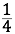PROPER FRACTION—a fraction whose numerator is smaller than the denominator, e.g.,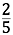IMPROPER FRACTION—a fraction whose numerator is larger than the denominator, e.g.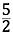MIXED FRACTION, also known as MIXED NUMBER—a combination of a WHOLE NUMBER and a PROPER FRACTION, e.g., ﻿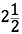DECIMAL—a fraction whose denominator is a power of ten and whose numerator consists of the digits following the DECIMAL POINT (dot or period), e.g., 0.4 (equivalent to ﻿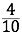) PERCENT—a decimal number multiplied by 100 and suffixed with the percent sign (%), e.g., 40% EQUIVALENT FRACTIONS—two or more fractions whose numerators and denominators are different, but give the same value when simplified LOWEST TERMS—the simplified form of a fraction

## Here are different ways of representing a fraction: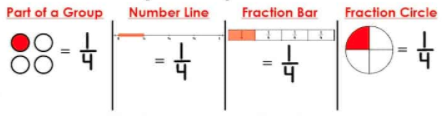## SIMPLIFYING FRACTIONS

The process of simplifying fractions consists in dividing both numerator and denominator of the fractions by the GREATEST COMMON FACTOR or GCF. Examples: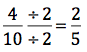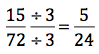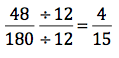Below is a set of problems on simplifying fractions.